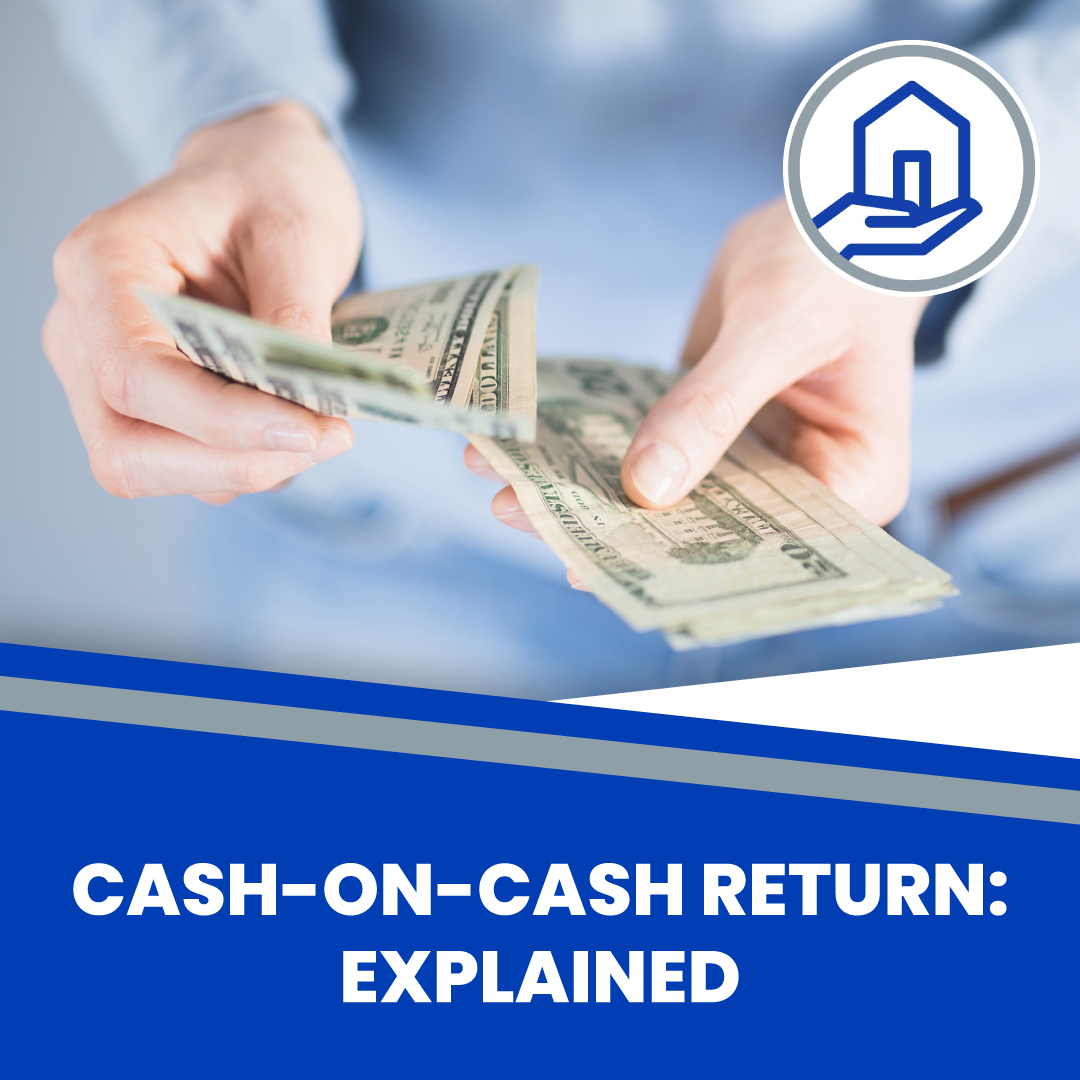# Cash-on-Cash Return: ExplainedIn an investment like real estate, cash flow can come in two forms rent and selling equity. CCR measures the cash generated from the property over an initial investment. Unlike the IRR, the CCR does not specify when funds will be reinvested. Here we will discuss the concept of Cash-on-Cash Return in Real Estate Investment.

What is Cash-on-Cash Return?

Cash-on-cash return is a financial term that refers to the ratio of cash flow generated by an investment over an initial investment. In other words, Cash-on-cash return is the ratio of total cash flow divided by the initial investment. The returns derived from cash-on-cash investments are generally low, and the investor would solely pay interest and finance charges on the borrowed funds. The CCR helps to determine the actual return that would be received over a period of time.

How do I calculate Cash-on-Cash Return?

The cash flow of an investment property is the difference between the net operating income and cash needed to pay expenses, debt service, and property taxes. A positive cash flow indicates that the operation generated a profit. Cash-on-Cash Return can be calculated in two different ways. The first method measures cash flow yields at the beginning and end of an investment. The second method is more relevant because it measures an asset’s starting to a specified period and shows how the initial investment was used to generate income for different periods on an annual basis.

How to evaluate Cash-on-Cash Return?

To evaluate the investment property’s cash flow, you need to determine the returns based on the initial investment. The percentage return is calculated by dividing net operating income by the initial investment. It can be considered sustainable if an investor earns a greater than 10 percent return annually on a property and keeps reinvesting the cash flow. If the investor has attained significant returns from their initial investment and does not have to sell equity to generate income.

Cash-on-Cash Return Formula

The cash-on-cash return formula lets the investor compute how much rent can be collected from a property with a certain amount of money invested. To calculate the return, the investor must know the property’s net operating income and initial investment. The net operating income is the difference between operating expenses and gross rent. The initial investment is the amount of money initially put into the property. The investor must know these items before calculating the cash-on-cash return formula.

Difference Between CoC & Other Return Calculations

The cash-on-cash return of a property is different from the other return calculations. The net operating income is the difference between gross rent and all expenses (including taxes, maintenance, and repair). The CoC formula measures how long it takes to pay off the initial investment. The time it takes to pay back the asset is represented in years. The return is calculated for one year or longer, depending on the period used by the property. Cash-on-cash return is also different from net operating income, which considers all rental property’s operating expenses.

A large number of investors have used the concept of cash flow return as a means to evaluate real estate investments. However, it does not provide the complete picture of investment. Analysts should be careful about not being overly optimistic about cash flow returns because it could hold investors back from deciding on whether to invest in the first place.